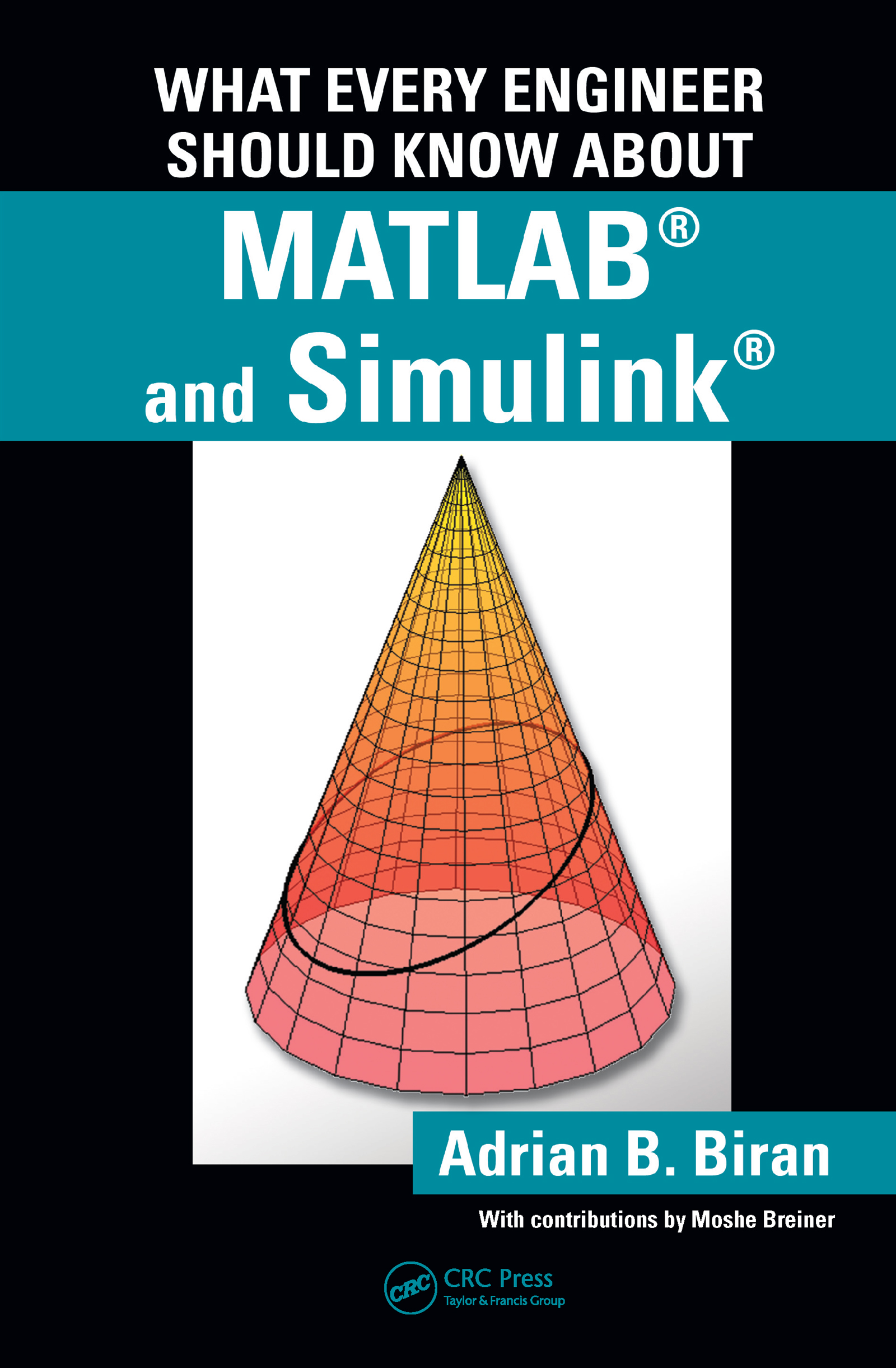What Every Engineer Should Know about MATLAB® and Simulink®

1st Edition

CRC Press

451 pages | 158 B/W Illus.

Purchasing Options:\$ = USD
Paperback: 9781439810200
pub: 2010-07-20
SAVE ~\$18.19
Currently out of stock
\$90.95
\$72.76
x
Hardback: 9781138422124
pub: 2017-07-27
SAVE ~\$41.00
\$205.00
\$164.00
x
eBook (VitalSource) : 9780429130717
pub: 2010-07-20
from \$43.98

FREE Standard Shipping!

Description

MATLAB® can be used to execute many mathematical and engineering calculations, as well as a handheld computer can—if not better. Moreover, like many other computer languages, it can perform tasks that a handheld computer cannot. Compared to other computer languages, MATLAB provides many built-in functions that make learning easier and reduce prototyping time. Simulink® is a toolbox that extends the possibilities of MATLAB by providing a graphical interface for modeling and simulating dynamical processes.

Using examples from mathematics, mechanical and electrical engineering, and control and signal processing, What Every Engineer Should Know About MATLAB® and Simulink® provides an introduction to these two computer environments and examines the advantages and limitations of MATLAB. It first explores the benefits of how to use MATLAB to solve problems and then process and present calculations and experimental results. This book also briefly introduces the reader to more advanced features of the software, such as object-oriented programming (OOP), and it draws the attention to some specialized toolboxes.

Key features of the book include demonstrations of how to:

• Visualize the results of calculations in various kinds of graphical representations
• Write useful script files and functions for solving specific problems
• Avoid disastrous computational errors
• Convert calculations into technical reports and insert calculations and graphs into either MS Word or LaTeX

This book illustrates the limitations of the computer, as well as the implications associated with errors that can result from approximations or numerical errors. Using selected examples of computer-aided errors, the author explains that the set of computer numbers is discrete and bounded—a feature that can cause catastrophic errors if not properly taken into account. In conjunction with The Mathworks—marketers of MATLAB and Simulink—a supplementary website is presented to offer access to software implemented in the book and the script files used to produce the figures. This book was written by Adrian B. Biran of Technion -- Israel Institute of Technology, with contributions by Moshe Breiner, managing director of SimACon.

I: Introducing MATLAB®

Introduction to MATLAB®

Starting MATLAB

Using MATLAB as a simple calculator

How to quit MATLAB

Using MATLAB as a scientific calculator

Arrays of numbers

Using MATLAB for plotting

Format

Arrays of numbers

Writing simple functions in MATLAB

Vectors and matrices

Vectors in geometry

Vectors in mechanics

Matrices

Matrices in geometry

Transformations

Matrices in Mechanics

Equations

Introduction

Linear equations in geometry

Linear equations in statics

Linear equations in electricity

On the solution of linear equations

Summary 1

More exercises

Polynomial equations

Iterative solution of equations

Processing and publishing the results

Copy and paste

Diary

Exporting and processing figures

Interpolation

The MATLAB spline function

Importing data from Excel – histograms

II: Programming in MATLAB®

Some facts about numerical computing

Introduction

Computer-aided mistakes

Computer representation of numbers

The set of computer numbers

Roundoff

Roundoff errors

Computer arithmetic

Why the examples in Section 5.2 failed

Truncation error

Complexity

Horner’s scheme

Problems that cannot be solved

Data types and object-oriented programming

Structures

Cell arrays

Classes and object-oriented programming

III: Progressing in MATLAB®

Complex numbers

The introduction of complex numbers

Complex numbers in MATLAB

Geometric representation

Trigonometric representation

Exponential representation

Functions of complex variables

Conformal mapping

Phasors

An application in mechanical engineering — a mechanism

Numerical integration

Introduction

The trapezoidal rule

Simpson’s rule

The MATLAB quadl function

Symbolic calculation of integrals

Ordinary differential equations

Introduction

Numerical solution of ordinary differential equations

Numerical solution of ordinary differential equations

Alternative strategies to solve ordinary differential equations

Conclusion: how to choose the codesolver

More graphics

Introduction

Drawing at scale

The cone surface and conic sections

GUIs - graphical user interfaces

An introduction to Simulink®

What is simulation

Beats

A model of the momentum law

Capacitor discharge

A mass-spring-dashpot system

A series RLC circuit

The pendulum

Applications in the frequency domain

Introduction

Signals

A short introduction to the DFT

The power spectrum

Trigonometric expansion of a signal

High frequency signals and aliasing

Bode plot

Answers to selected questions

Bibliography

Index

Adrian B. Biran is on the faculty of mechanical engineering at the Technion—Israel Institute of Technology. He received his MSc and DSc from that same school, as well as a Diplomat Engineer degree from the Bucharest Polytechnic Institute. He worked extensively in design in Romania at IPRONAV—The Institute of Ship Projects, the Bucharest Studios and IPA—The Institute of Automation Projects. In Israel, he worked in design at the Israel Shipyards, and in research on Naval Architectural subjects at the Technion Research and Development Foundation. In parallel, he worked as a project instructor in Romania at the Technical Military Academy, in Israel at the Beer Sheva University (now the Ben Gurion University). Since 1972, Biran has served as an adjunct teacher in the Faculty of Mechanical Engineering of the Technion, and for the last 15 years as Adjunct Associate Professor. He has taught subjects including Machine Design, Engineering Drawing, and especially Naval Architecture. He has authored several papers on subjects such as computational linguistics and computer simulations of marine systems and subjects belonging to Ship Design. He also wrote a book on ships for popular audience and a book on Ship Hydrostatics and Stability published in English and Turkish. Together with Moshe Breiner he wrote a book on MATLAB® for Engineers that was published in three English, three German, two French, and two Greek editions.

Moshe Breiner graduated from the Scuola Normale di Pisa and the Universitá degli Studi di Pisa and obtained a Ph.D degree from the Harvard Graduate School of Arts and Sciences. He has worked in modeling and simulations and taught MATLAB®.